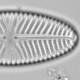## Please archive assemblage data as counts not percent

A large fraction of the microfossil assemblage that has been archived on-line by palaeoecologists is percent data, often without the count sums, rather than the raw count data. This is unfortunate as some analyses need count data. Calculating percent from count data is trivial, but the reverse operation is in general not possible if the count sums are not available.

Fortunately, one aspect of assemblage data makes it possible to estimate the count sums: the long tail of most rank-abundance distributions means that the rarest taxon in any sample is probably present with a single individual. The lowest percent abundance,$P_{min}$, is therefore$1/count * 100$ and the count sum can be estimated as$100/P_{min}$.

Archived data are often rounded off which limits the precision of this calculation, but it is possible to estimate the range of possible count sums using the maximum and minimum value of$P_{min}$ that would yield the reported$P_{min}$ after rounding. Obviously, the uncertainty increases when fewer decimal places are reported, and with larger count sums.

I’ve written an R function to estimate count sums in the R package `countChecker` which is available on Github.

```#devtools::install_github("richardjtelford/count_checker")
library("countChecker")
library("tidyverse")
```

Lets see it in action with the percent data from Last Chance Lake (Axford et al 2017), chosen because I like the name. More importantly, it was the first percent assemblage data I found on NOAA were the count sums were reported, so it is possible to check the code is working.

```#Import data
data("last_chance")

#examine data to determine precision
```
``````## Cric.pulTy Cric.Orthund Cric.sylTy Cric.treTy
## 1 0.00 3.53 0.00 0.00
## 2 0.85 6.78 0.00 1.69
## 3 0.00 1.92 0.00 0.00
## 4 0.25 0.98 0.49 1.47
## 5 0.00 2.26 0.75 2.26
## 6 0.00 0.00 0.00 4.57``````
```#Isolate species data
last_chance = select(last_chance0, -age_calBP, -totcaps)

#Estimate n and add reported n
last_chance_est = estimate_n(spp = last_chance, digits = 2) %>%
mutate(n = last_chance0\$totcaps)

#plot
last_chance_est %>%
ggplot(aes(x = n, y = est_n, ymax = est_max, ymin = est_min)) +
geom_pointrange() +
geom_abline(
aes(slope = slope, intercept = 0, colour = as.factor(slope)),
data = data_frame(slope = 1:2),
show.legend = FALSE) +
labs(x = "Count sum", y = "Estimated count sum")
```Estimated vs reported count sums for Last Chance Lake. The lower line is the 1:1 relationship. The upper line is the 2:1 relationship expected when the rarest taxon is represented by a half chironomid.

This reveals both the power of this method, and one of a few problems. A few samples have estimated counts that match the reported counts, but most have estimated counts twice the reported counts. The problem is that chironomid workers often count half head capsules: in many samples the lowest abundance is a half chironomid. Pollen and occasionally diatoms are also sometimes counted with halves – integer counts are easier to analyse.

In other datasets, there might be some samples with the rarest taxon represented by two (or more) microfossils. This is probably only a serious risk in very low diversity systems (hello Neogloboquadrina pachyderma sinistral), or where only a few common taxa are identified rather than all taxa.

Diatoms present an interesting test case as the two valves which comprise the frustule are counted separately even though they are often found paired (or in longer chains), raising the risk that the rarest taxon has two valves. If the rarest taxon has two valves, it is unlikely that the other taxa all have multiples of two valves, which should help detect this problem which would otherwise make the count appear to be half its true size.

The first diatom assemblage dataset I found on pangaea.de appeared to have a few samples with count sums much lower than reported, even allowing for the possibility that the rarest taxon is not a singleton. This is not the first time that I’ve noticed that some authors appear to report how many microfossils they planned to count rather than the number they actually counted. This is difficult to detect with percent data but `countChecker` might reveal it. I plan, at some stage, to trawl through various archived assemblage data to test how common this problem is.

The `countChecker` package also tests whether the percent data are congruent using a method very similar to the GRIM test which has been used to great effect on psycology data. The principle is this: if the count is of$n$ microfossils, then, discounting the tendency of some analysts to count fractional microfossils, only integer multiples of$100/n$ are possible. The `percent_checker` function tests for such impossible values after allowing for limited precision in both the percent data and the count sum. I’ll explore this method more later.

Pollen data archived in Neotoma and the European Pollen Database are nearly all count data. Please can other microfossil assemblage data also be archived as counts not percent.